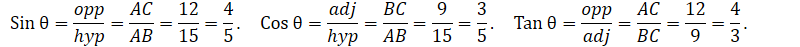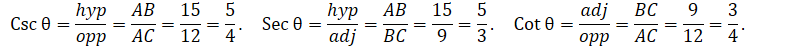It looks like you're using Internet Explorer 11 or older. This website works best with modern browsers such as the latest versions of Chrome, Firefox, Safari, and Edge. If you continue with this browser, you may see unexpected results.

# Math

## Trigonometric Ratios and Pythagorean Theorem

##### 1) Sine of an angle: In a right triangle, the sine or sin of an angle is the ratio of the side opposite to the angle to the hypotenuse:##### 2) The Cosine of an angle: In a right triangle, the cosine or cos of an angle is defined as the ratio of the side adjacent to an acute angle to the hypotenuse.##### 3) Tangent of an angle: Tangent or Tan of an angle is defined as the ratio of the side opposite to an angle to the side adjacent to the angle.##### 4) Cotangent of an angle: Cotangent or Cot of an angle is defined as the ratio of the side adjacent to an angle to the side opposite to the angle.##### 5) Cosecant of an angle: Cosecant or Csc of an angle is defined as the ratio of the hypotenuse to the side opposite to the angle.########## Example: Find the trig ratios of the given angle.##### Example: In the triangle shown below, find the value of x.## Pythagorean Theorem

##### The Pythagorean theorem states a fundamental relationship between three sides of a right triangle. According to this theorem, the area of a square whose side is the hypotenuse  is equal to the sum of the areas of the squares whose sides are the other two sides of a right triangle.##### Example: Find the unknown side of the triangle below.##### We use the Pythagorean theorem to find c: